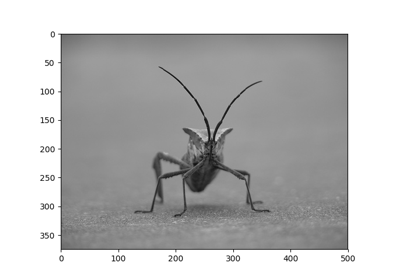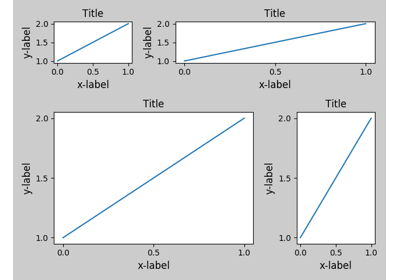# matplotlib.pyplot.imshow¶

`matplotlib.pyplot.``imshow`(X, cmap=None, norm=None, aspect=None, interpolation=None, alpha=None, vmin=None, vmax=None, origin=None, extent=None, shape=None, filternorm=1, filterrad=4.0, imlim=None, resample=None, url=None, hold=None, data=None, **kwargs)

Display an image on the axes.

Parameters: Returns: X : array_like, shape (n, m) or (n, m, 3) or (n, m, 4) Display the image in `X` to current axes. `X` may be an array or a PIL image. If `X` is an array, it can have the following shapes and types: MxN – values to be mapped (float or int) MxNx3 – RGB (float or uint8) MxNx4 – RGBA (float or uint8) MxN arrays are mapped to colors based on the `norm` (mapping scalar to scalar) and the `cmap` (mapping the normed scalar to a color). Elements of RGB and RGBA arrays represent pixels of an MxN image. All values should be in the range [0 .. 1] for floats or [0 .. 255] for integers. Out-of-range values will be clipped to these bounds. cmap : `Colormap`, optional, default: None If None, default to rc `image.cmap` value. `cmap` is ignored if `X` is 3-D, directly specifying RGB(A) values. aspect : [‘auto’ | ‘equal’ | scalar], optional, default: None If ‘auto’, changes the image aspect ratio to match that of the axes. If ‘equal’, and `extent` is None, changes the axes aspect ratio to match that of the image. If `extent` is not `None`, the axes aspect ratio is changed to match that of the extent. If None, default to rc `image.aspect` value. interpolation : string, optional, default: None Acceptable values are ‘none’, ‘nearest’, ‘bilinear’, ‘bicubic’, ‘spline16’, ‘spline36’, ‘hanning’, ‘hamming’, ‘hermite’, ‘kaiser’, ‘quadric’, ‘catrom’, ‘gaussian’, ‘bessel’, ‘mitchell’, ‘sinc’, ‘lanczos’ If `interpolation` is None, default to rc `image.interpolation`. See also the `filternorm` and `filterrad` parameters. If `interpolation` is ‘none’, then no interpolation is performed on the Agg, ps and pdf backends. Other backends will fall back to ‘nearest’. norm : `Normalize`, optional, default: None A `Normalize` instance is used to scale a 2-D float `X` input to the (0, 1) range for input to the `cmap`. If `norm` is None, use the default func:`normalize`. If `norm` is an instance of `NoNorm`, `X` must be an array of integers that index directly into the lookup table of the `cmap`. vmin, vmax : scalar, optional, default: None `vmin` and `vmax` are used in conjunction with norm to normalize luminance data. Note if you pass a `norm` instance, your settings for `vmin` and `vmax` will be ignored. alpha : scalar, optional, default: None The alpha blending value, between 0 (transparent) and 1 (opaque). The `alpha` argument is ignored for RGBA input data. origin : [‘upper’ | ‘lower’], optional, default: None Place the [0,0] index of the array in the upper left or lower left corner of the axes. If None, default to rc `image.origin`. extent : scalars (left, right, bottom, top), optional, default: None The location, in data-coordinates, of the lower-left and upper-right corners. If `None`, the image is positioned such that the pixel centers fall on zero-based (row, column) indices. shape : scalars (columns, rows), optional, default: None For raw buffer images filternorm : scalar, optional, default: 1 A parameter for the antigrain image resize filter. From the antigrain documentation, if `filternorm` = 1, the filter normalizes integer values and corrects the rounding errors. It doesn’t do anything with the source floating point values, it corrects only integers according to the rule of 1.0 which means that any sum of pixel weights must be equal to 1.0. So, the filter function must produce a graph of the proper shape. filterrad : scalar, optional, default: 4.0 The filter radius for filters that have a radius parameter, i.e. when interpolation is one of: ‘sinc’, ‘lanczos’ or ‘blackman’ image : `AxesImage` **kwargs : `Artist` properties.

`matshow`
Plot a matrix or an array as an image.

Notes

Unless extent is used, pixel centers will be located at integer coordinates. In other words: the origin will coincide with the center of pixel (0, 0).

Two typical representations are used for RGB images with an alpha channel:

• Straight (unassociated) alpha: R, G, and B channels represent the color of the pixel, disregarding its opacity.
• Premultiplied (associated) alpha: R, G, and B channels represent the color of the pixel, adjusted for its opacity by multiplication.

`imshow` expects RGB images adopting the straight (unassociated) alpha representation.

Note

In addition to the above described arguments, this function can take a data keyword argument. If such a data argument is given, the following arguments are replaced by data[<arg>]:

• All positional and all keyword arguments.

## Examples using `matplotlib.pyplot.imshow`¶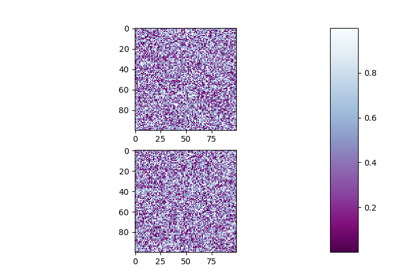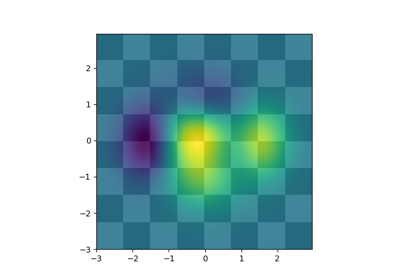Layer Images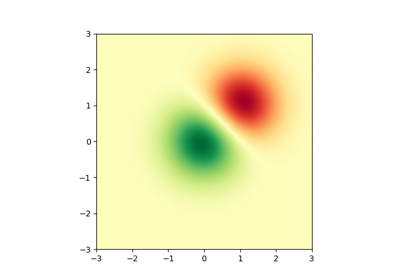Image Demo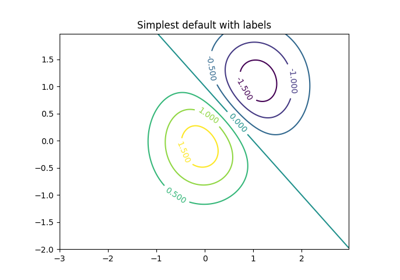Contour Demo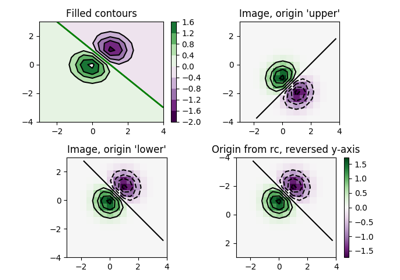Contour Image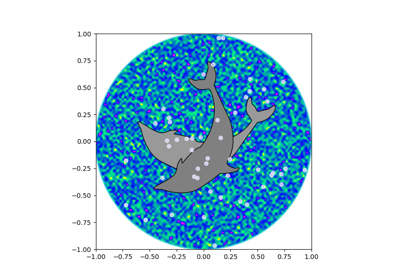Dolphins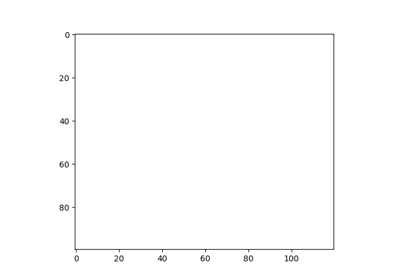Animated image using a precomputed list of images